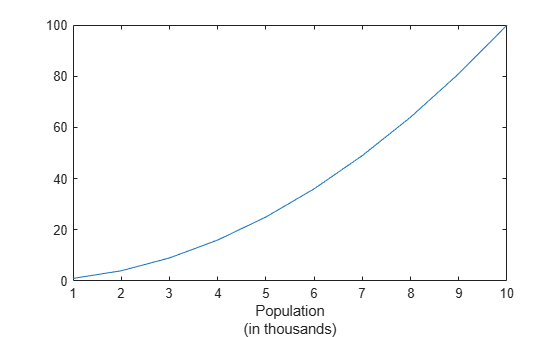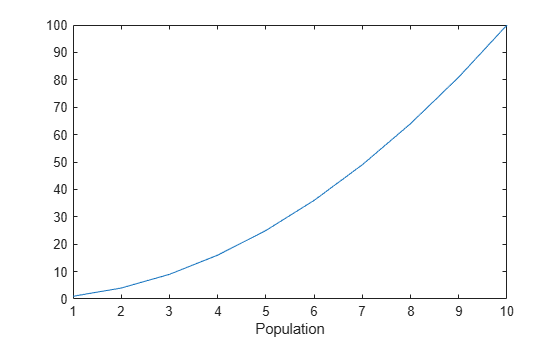# xlabel

## Syntax

xlabel(txt)
xlabel(target,txt)
xlabel(___,Name,Value)
t = xlabel(___)

## Description

example

xlabel(txt) labels the x-axis of the current axes or standalone visualization. Reissuing the xlabel command replaces the old label with the new label.

example

xlabel(target,txt) adds the label to the specified target object.

example

xlabel(___,Name,Value) modifies the label appearance using one or more name-value pair arguments. For example, 'FontSize',12 sets the font size to 12 points. Specify name-value pair arguments after all other input arguments. Modifying the label appearance is not supported for all types of charts. 

example

t = xlabel(___) returns the text object used as the x-axis label. Use t to make future modifications to the label after it is created.

## Examples

collapse all

Display Population beneath the x-axis.

plot((1:10).^2) xlabel('Population')Create a multiline label using a cell array of character vectors.

plot((1:10).^2) xlabel({'Population','(in thousands)'})Include Greek letters and other special characters in the label using TeX markup.

x = linspace(-2*pi,2*pi); y = sin(x); plot(x,y) xlabel('-2\pi \leq x \leq 2\pi')Add a label with text and a variable value. Use the num2str function to include a variable value in the label.

plot((1:10).^2) year = 2014; xlabel(['Population for Year ',num2str(year)])Use the '^' and '_' characters to include superscripts and subscripts in the axis labels. Use curly braces {} to modify more than one character.

t = linspace(0,1); y = exp(t); plot(t,y) xlabel('t_{seconds}') ylabel('e^t')Use Name,Value pair arguments to set the font size, font weight, and text color properties of the x-axis label.

plot((1:10).^2) xlabel('Population','FontSize',12,'FontWeight','bold','Color','r')Starting in R2019b, you can display a tiling of plots using the tiledlayout and nexttile functions. Call the tiledlayout function to create a 2-by-1 tiled chart layout. Call the nexttile function to create the axes objects ax1 and ax2. Plot data into each axes, and create an x-axis label for the top plot.

tiledlayout(2,1) ax1 = nexttile; plot((1:10).^2) xlabel(ax1,'Population') ax2 = nexttile; plot((1:10).^3)Label the x-axis and return the text object used as the label.

plot((1:10).^2) t = xlabel('Population');Use t to set text properties of the label after it has been created. For example, set the color of the label to red. Use dot notation to set properties.

t.Color = 'red';## Input Arguments

collapse all

Axis label, specified as a string scalar, character vector, string array, character array, cell array, categorical array, or numeric value.

Example: 'my label'

Example: {'first line','second line'}

Example: 123

To include numeric variables with text in a label, use the num2str function. For example:

x = 42; txt = ['The value is ',num2str(x)];

To include special characters, such as superscripts, subscripts, Greek letters, or mathematical symbols use TeX markup. For a list of supported markup, see the Interpreter property.

To create multiline labels:

• Use a string array, where each element contains a line of text, such as ["first line","second line"].

• Use a cell array, where each cell contains a line of text, such as {'first line','second line'}.

• Use a character array, where each row contains the same number of characters, such as ['abc'; 'ab '].

• Use sprintf to create text with a new line character, such as sprintf('first line \n second line').

Numeric labels are converted to text using sprintf('%g',value). For example, 12345678 displays as 1.23457e+07.

Note

• If you specify the label as a categorical array, MATLAB® uses the values in the array, not the categories.

• The words default, factory, and remove are reserved words that will not appear in a label when quoted as a normal characters. To display any of these words individually, precede them with a backslash, such as '\default' or '\remove'.

Target for label, specified as one of the following:

• An Axes object.

• A TiledChartLayout object.

• A standalone visualization that has an XLabel property. For example, you can specify a heatmap chart as the target.

• An array of graphics objects from the preceding list. The objects must belong to the same class. To determine the class, use the class function.

If you do not specify the target, then the xlabel function adds the label to the graphics object returned by the gca command.

Note

Standalone visualizations do not support modifying the label appearance, such as the color, or returning the text object as an output argument.

### Name-Value Arguments

Specify optional pairs of arguments as Name1=Value1,...,NameN=ValueN, where Name is the argument name and Value is the corresponding value. Name-value arguments must appear after other arguments, but the order of the pairs does not matter.

Before R2021a, use commas to separate each name and value, and enclose Name in quotes.

Example: 'Color','red','FontSize',12 specifies red, 12-point font.

The text properties listed here are only a subset. For a complete list, see Text Properties.

Font size, specified as a scalar value greater than 0 in point units. One point equals 1/72 inch. To change the font units, use the FontUnits property.

Setting the font size properties for the associated axes also affects the label font size. The label font size updates to equal the axes font size times the label scale factor. The FontSize property of the axes contains the axes font size. The LabelFontSizeMultiplier property of the axes contains the label scale factor. By default, the axes font size is 10 points and the scale factor is 1.1, so the x-axis label font size is 11 points.

Data Types: single | double | int8 | int16 | int32 | int64 | uint8 | uint16 | uint32 | uint64

Character thickness, specified as 'normal' or 'bold'.

MATLAB uses the FontWeight property to select a font from those available on your system. Not all fonts have a bold weight. Therefore, specifying a bold font weight can still result in the normal font weight.

Font name, specified as a supported font name or 'FixedWidth'. To display and print text properly, you must choose a font that your system supports. The default font depends on your operating system and locale.

To use a fixed-width font that looks good in any locale, use 'FixedWidth'. The fixed-width font relies on the root FixedWidthFontName property. Setting the root FixedWidthFontName property causes an immediate update of the display to use the new font.

Text color, specified as an RGB triplet, a hexadecimal color code, a color name, or a short name.

For a custom color, specify an RGB triplet or a hexadecimal color code.

• An RGB triplet is a three-element row vector whose elements specify the intensities of the red, green, and blue components of the color. The intensities must be in the range [0,1]; for example, [0.4 0.6 0.7].

• A hexadecimal color code is a character vector or a string scalar that starts with a hash symbol (#) followed by three or six hexadecimal digits, which can range from 0 to F. The values are not case sensitive. Thus, the color codes '#FF8800', '#ff8800', '#F80', and '#f80' are equivalent.

Alternatively, you can specify some common colors by name. This table lists the named color options, the equivalent RGB triplets, and hexadecimal color codes.

Color NameShort NameRGB TripletHexadecimal Color CodeAppearance
'red''r'[1 0 0]'#FF0000''green''g'[0 1 0]'#00FF00''blue''b'[0 0 1]'#0000FF''cyan' 'c'[0 1 1]'#00FFFF''magenta''m'[1 0 1]'#FF00FF''yellow''y'[1 1 0]'#FFFF00''black''k'[0 0 0]'#000000''white''w'[1 1 1]'#FFFFFF''none'Not applicableNot applicableNot applicableNo color

Here are the RGB triplets and hexadecimal color codes for the default colors MATLAB uses in many types of plots.

[0 0.4470 0.7410]'#0072BD'[0.8500 0.3250 0.0980]'#D95319'[0.9290 0.6940 0.1250]'#EDB120'[0.4940 0.1840 0.5560]'#7E2F8E'[0.4660 0.6740 0.1880]'#77AC30'[0.3010 0.7450 0.9330]'#4DBEEE'[0.6350 0.0780 0.1840]'#A2142F'Example: 'blue'

Example: [0 0 1]

Example: '#0000FF'

Text interpreter, specified as one of these values:

• 'tex' — Interpret characters using a subset of TeX markup.

• 'latex' — Interpret characters using LaTeX markup.

• 'none' — Display literal characters.

#### TeX Markup

By default, MATLAB supports a subset of TeX markup. Use TeX markup to add superscripts and subscripts, modify the font type and color, and include special characters in the text.

Modifiers remain in effect until the end of the text. Superscripts and subscripts are an exception because they modify only the next character or the characters within the curly braces. When you set the interpreter to 'tex', the supported modifiers are as follows.

ModifierDescriptionExample
^{ }Superscript'text^{superscript}'
_{ }Subscript'text_{subscript}'
\bfBold font'\bf text'
\itItalic font'\it text'
\slOblique font (usually the same as italic font)'\sl text'
\rmNormal font'\rm text'
\fontname{specifier}Font name — Replace specifier with the name of a font family. You can use this in combination with other modifiers.'\fontname{Courier} text'
\fontsize{specifier}Font size —Replace specifier with a numeric scalar value in point units.'\fontsize{15} text'
\color{specifier}Font color — Replace specifier with one of these colors: red, green, yellow, magenta, blue, black, white, gray, darkGreen, orange, or lightBlue.'\color{magenta} text'
\color[rgb]{specifier}Custom font color — Replace specifier with a three-element RGB triplet.'\color[rgb]{0,0.5,0.5} text'

This table lists the supported special characters for the 'tex' interpreter.

Character SequenceSymbolCharacter SequenceSymbolCharacter SequenceSymbol

\alpha

α

\upsilon

υ

\sim

~

\angle

\phi

ϕ

\leq

\ast

*

\chi

χ

\infty

\beta

β

\psi

ψ

\clubsuit

\gamma

γ

\omega

ω

\diamondsuit

\delta

δ

\Gamma

Γ

\heartsuit

\epsilon

ϵ

\Delta

Δ

\spadesuit

\zeta

ζ

\Theta

Θ

\leftrightarrow

\eta

η

\Lambda

Λ

\leftarrow

\theta

θ

\Xi

Ξ

\Leftarrow

\vartheta

ϑ

\Pi

Π

\uparrow

\iota

ι

\Sigma

Σ

\rightarrow

\kappa

κ

\Upsilon

ϒ

\Rightarrow

\lambda

λ

\Phi

Φ

\downarrow

\mu

µ

\Psi

Ψ

\circ

º

\nu

ν

\Omega

Ω

\pm

±

\xi

ξ

\forall

\geq

\pi

π

\exists

\propto

\rho

ρ

\ni

\partial

\sigma

σ

\cong

\bullet

\varsigma

ς

\approx

\div

÷

\tau

τ

\Re

\neq

\equiv

\oplus

\aleph

\Im

\cup

\wp

\otimes

\subseteq

\oslash

\cap

\in

\supseteq

\supset

\lceil

\subset

\int

\cdot

·

\o

ο

\rfloor

\neg

¬

\nabla

\lfloor

\times

x

\ldots

...

\perp

\surd

\prime

´

\wedge

\varpi

ϖ

\0

\rceil

\rangle

\mid

|

\vee

\langle

\copyright

#### LaTeX Markup

To use LaTeX markup, set the interpreter to 'latex'. For inline mode, surround the markup with single dollar signs ($). For display mode, surround the markup with double dollar signs ($$). LaTeX ModeExampleResult Inline '\int_1^{20} x^2 dx'Display '$$\int_1^{20} x^2 dx$\$'The displayed text uses the default LaTeX font style. The FontName, FontWeight, and FontAngle properties do not have an effect. To change the font style, use LaTeX markup.

The maximum size of the text that you can use with the LaTeX interpreter is 1200 characters. For multiline text, this reduces by about 10 characters per line.

For examples that use TeX and LaTeX, see Greek Letters and Special Characters in Chart Text. For more information about the LaTeX system, see The LaTeX Project website at https://www.latex-project.org/.

## Output Arguments

collapse all

Text object used as the x-axis label. Use t to access and modify properties of the label after it has been created.

## Tips

• By default, the Interactions property contains editInteraction so the text can be edited by clicking on the text. To disable this interaction, set the Interactions property of the text object to [].

## Version History

Introduced before R2006a